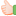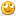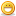1. ## boundary value analysis

Hi,

What is boundary value analysis and equivalence partitioning method with example.
Thanks.Reply With Quote

2. ##Re: boundary value analysis

Hi,

Consider a situation where u pass the number as the input .(eg: Entering ur marks on a web page.)

Assumption: marks are between 0 to 100

For this
1) Boundary value analysis : take the boundaries 0 and 100 and test for the condition such as -1,0,+1 and 99,100,101

2) Equivalence partitioning : is not as such a stand alone method for testing. It is implemented by Boundary value analysis.

Partition the inputs into a) valid and b) invalid partition as
a) valid 0 -100
b) invalid -1 to -infinity and 101 to infinity

and then apply the boundary value analysis for the above.

Still if u not clear, use this Equivalence partitioning, Boundary value analysis

Regards,
Elango.Reply With Quote

3. ## Re: boundary value analysis

what r the possible test cases for railway reservation systemReply With Quote

4. ## Re: boundary value analysis

Boundary value analysis is one of the method of black box testing, in which the values on the boundary is tested. For example, if the benefit amount should fall in the range \$1000 to \$10000, the testing on the boundary will be carried based on the following inputs. I. On the boundary - \$1000 and \$10000, the test case should pass ii. Below the boundary - value less than \$1000, the test case should fail ii. Above the boundary - value greater than \$10000, the test case should fail equivalence partitioning is another approach of testing followed in black box testing where the input values are partioned and tested. For example, if the benefit amount is in the range \$1000 to \$10000 then the following criterias are tested as the input values are equally partioned in to three categories. I. Values less than \$1000, the test case should fail ii. Values between \$1000 to \$10000 the test case should pass iii. Values greater than \$10000 the test case should fail warm regards, ganesanReply With Quote

5. ##Re: boundary value analysis

Boundary value analysis:a test data selection technique in which values are chosen to lie along data extremes. design the test cases to validate max,mini values outside/inside ,typical and error values by the system.
Equivalance partition:design the testcases to validate the application for valid and invalid testdata.Reply With Quote

6. ##Re: boundary value analysis

Hi bagavathi ! Boundary value analysis is testing the boundary condiitions.
Ex : If you have to test the functionality of A prime number prog. working on numbers between 1 to 1000.
Then your test conditions will be :
BVA :
0
1
2
999
1000
1001
U have to check the behaviour of the prog. with the numbers excatly at the boundary, imm. outside the boundary, imm. inside the boundary.

Equivalance partition :
It has got three parts :

(1) Input partitions : The set of inputs can be partitioned into similar type of data to avoid exhaustive testing.
For example: consider a program
which accepts any value between
-10000 and +10000.
Then equivalence partition would be;
Less than zero,
Zero &
Greater than zero
(2) Output partitions : Even the output values can be partitioned just like inputs.
For example: A university declares a result of its student as Fail if the score is 0-35, 2nd class for 35-60, 1st class for 60-75 and distinction for 75 and above. Then o/p partition would be;
0-35 Fail
35-60 second class
60-75 first class
75 and above distinction
(3)Other partitions : It is used when we know enough about application and is an optional on. This is more technical and difficultone.

Regards,
Saurabh GargReply With Quote

####Posting Permissions

• You may not post new threads
• You may not post replies
• You may not post attachments
• You may not edit your posts
•